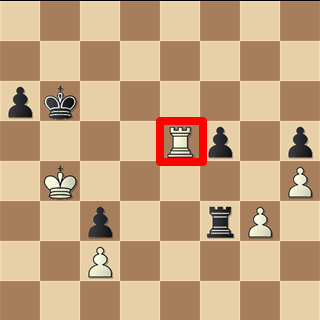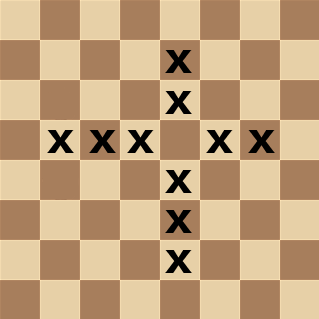# Generating Magic Multipliers

I finally cracked the process needed to generate magic multipliers.  I didn’t find it trivial.  Here are necessary steps:

## 1. Create Combination Table:

When generating magic moves the first step is to logically AND the board with the relevant square’s mask.  Let’s use the rook on e5 in the diagram below as an example:Note the edge squares are not part of the mask. The reason is simple.  For example, if the rook can move to e2 it can always attack, protect or move  to e1.  This trick also reduced the bits in the mask; an important point as we’ll see in a moment.

So the bitboard after the AND operation only has one bit set, corresponding to the black pawn on f5.  We’ll call this the AND_RESULT.  In this case it look like this:After the magic operation this will provide a look-up to the possible attacking moves of the rook.  We’ll call this the ROOK_ATTACKS.  In this case it is the following bitboard:There are a couple of things to note:

• If g5 was set in the AND_RESULT we’d still have the same ROOK_ATTACKS
• There are (2 to the power BitCount) possible AND_RESULTs, where BitCount is the number of bits set in the AND_MASK.

So now we need to create a list of all the possible AND_RESULTs as well the corresponding ROOK_ATTACKS.  Once again this isn’t trivial.  How do you create all of the possible permutation of the AND_RESULT?  The key is a little function which take as inputs the AND_MASK and the index you’d like to create (the index being between zero and 2 ^ BitCount).  Here’s the Delphi code I used:

```function TMagicGenerator.IndexTo64(AndMask: TBitboard; Index: integer): TBitboard; var i, n, b: integer; a: TBitboard; begin result := 0; a := AndMask; n := PopCount(a); for i := 0 to n − 1 do begin b := BSF_Reset(a); if IsBitSet(i, index) then SetBit(b, result); end; end;```

This loops through all of the bits set in AndMask.  Each bit has an index (in this case “i” which runs form 0 to n-1) and a position (in this case “b” which is between 0 and 63).  If index also has the “i” bit set then the “b” bit is also set in the result.  It’s worth spending a couple of minutes getting your head around this.  As I said it’s not trivial but nevertheless core to the whole approach.

The function BSF_Reset(a) returns the position of the first set bit and resets that bit.  The other functions are self explanatory.

Once we have this function we can create a list of all the possible AND_RESULTS and their corresponding ROOK_ATTACKS.

```procedure TMagicGenerator.CreateAllRookMasks(b: Tbitboard; square: integer); var n: integer; i: integer; AndMask, AttackMask: TBitboard; begin n := PopCount(b); Setlength(AndAttackMask, Round(Power(2, n))); for i := 0 to High(AndAttackMask) do begin AndAttackMask[i].AndMask := IndexTo64(b, i); AndAttackMask[i].AttackMask := CreateRookAttacks(square, AndAttackMask[i].AndMask); end; end;```

## 2. Test Candidate Magic Multipliers:

For a magic to be valid one of two condition must be met:

• The magic must map each AND_RESULT to a unique position in a look-up table

or

• The magic must map the AND_RESULTS to a position with the same ROOK_ATTACKS

This can be accomplished by:

1. Creating the look-up table
2. Setting all values to zero
3. Looping through all AND_RESULT transforming them into a look-up table index
4. Check to see if the look-up table already contains a ROOK_ATTACK
5. If it does have a ROOK_ATTACK already stored then it must be the same as the one corresponding to the AND_RESULT – if it isn’t the magic is not valid
6. The magic is valid if this sequence can be completed for all possible AND_RESULTs

The code I used for this is here:
```function TMagicGenerator.MagicWorks(xMagic: uint64): boolean; var i: integer; b: uint64; begin // Zero Test table for i := 0 to High(MagicTable) do MagicTable[i] := 0; // Test each possible mask result := true; i := High(AndAttackMask); repeat b := (AndAttackMask[i].AndMask * xMagic) shr (64 - RookBitCount); if MagicTable[b] = 0 then MagicTable[b] := AndAttackMask[i].AttackMask else if MagicTable[b] <> AndAttackMask[i].AttackMask then result := false; dec(i); until (result = false) or (i < 0); end; procedure TMagicGenerator.FindRookMagics(Trials: integer); var i, n: Integer; Square: integer; xMagic: uint64; RookMask: TBitboard; begin SetLength(MagicTable, Round(Power(2, RookBitCount))); // Initialize for Square := 0 to 63 do begin if assigned(fOnNewSquare) then fOnNewSquare(self, square); RookMask := CreateRookMask(Square); CreateAllRookMasks(RookMask, Square); for i := 0 to Trials do begin if (i and 1023) = 0 then Application.ProcessMessages; // Find a candidate magic repeat xMagic := SparseRandom64; until BitCount(xMagic) > 6; // Does Candidate work if MagicWorks(xMagic) then begin if assigned(fOnFoundMagic) then fOnFoundMagic(self, Square, xMagic); Break; end; end; if i > Trials then if assigned(fOnSquareFail) then fOnSquareFail(self, Square); end; end;```

Note the random magic number seems to work best with a sparse 64 bit integer.

What I’ve outlined is the simplest form of magic bitboard with a fixed shift.  One nice aspect of magic move generation is it’s a stand-alone part of the chess engine.  So any improvements to the approach (e.g. more compact look-up tables) can be added later.

A couple of resource I found helpful were Looking for Magic and Rival Chess’ Page